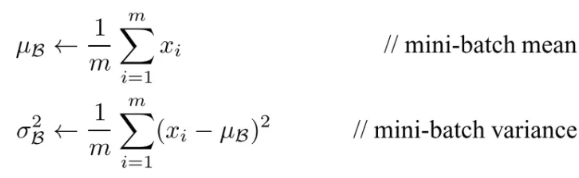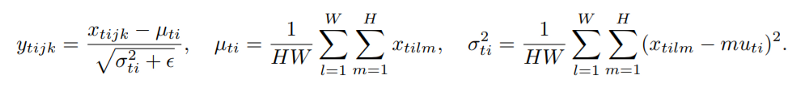2 views

I understand that Batch Normalisation helps in faster training by turning the activation towards unit Gaussian distribution and thus tackling vanishing gradients problem. Batch norm acts is applied differently at training(use mean/var from each batch) and test time (use finalized running mean/var from training phase).

Instance normalization, on the other hand, acts as contrast normalization as mentioned in this paper. The authors mention that the output stylized images should not depend on the contrast of the input content image and hence Instance normalization helps.

But then should we not also use instance normalization for image classification where the class label should not depend on the contrast of the input image. I have not seen any paper using instance normalization in-place of batch normalization for classification. What is the reason for that? Also, can and should batch and instance normalization be used together. I am eager to get an intuitive as well as a theoretical understanding of when to use which normalization.

by (33.1k points)

Batch Normalization

It is a method that normalizes activations in a network across the mini-batch of definite size. For each feature, batch normalization computes the mean and variance of that feature in the mini-batch. It then subtracts the mean and divides the feature by its mini-batch standard deviation.Instance Normalization

Instance normalization normalizes across each channel in each training example instead of normalizing across input features in a training example. Unlike batch normalization, the instance normalization layer is applied at test time as well(due to the non-dependency of mini-batch).Which normalization is better?

The answer depends on the network architecture, in particular on what is done after the normalization layer.

This is where the distribution refinements start to matter: the same neuron is going to receive the input from all images. If the variance across the batch is high, the gradient from the small activations will be completely suppressed by the high activations, which is exactly the problem that the batch norm tries to solve. That's why it's fairly possible that per-instance normalization won't improve network convergence at all.

On the other hand, batch normalization adds extra noise to the training, because the result for a particular instance depends on the neighbor instances. As it turns out, this kind of noise may be either good and bad for the network. This is well explained in the "Weight Normalization" paper by Tim Salimans et al, which name recurrent neural networks and reinforcement learning DQNs as noise-sensitive applications. I'm not entirely sure, but I think that the same noise-sensitivity was the main issue in the stylization task, which instance norm tried to fight. It would be interesting to check if the weight norm performs better for this particular task.

Can you combine batch and instance normalization?

Though it makes a valid neural network, there's no practical use for it. Batch normalization noise is either helping the learning process or hurting it. In both cases, leaving the network with one type of normalization is likely to improve the performance.